## 1. Introduction

In this tutorial, we’ll look at two types of merging algorithms: 2-way merge and-way merge, which are both highly significant. Furthermore, we’ll briefly go through two-way and-way merging, covering how they work, how to apply certain merge algorithms, and their time and space complexity.

## 2. Merge Algorithm

Merging is a process of combining two or more types of structures into one single structure, which is an important component of algorithms such as merge sort.

If we have two or generally more arrays, we can merge them and get a single array or list.

Practically speaking, the size of the merged array is the same as the total size of the merged arrays.

### 2.1. Sorted Merging

There are various types of merging methods.

Sorted merging simply combines the items of the structures that are already in sorted order.

Let’s see the following example. First, we take two or more sorted arrays and compare the first items.

The first elements of two sorted arrays are compared, and the smaller element is taken and added to the output array: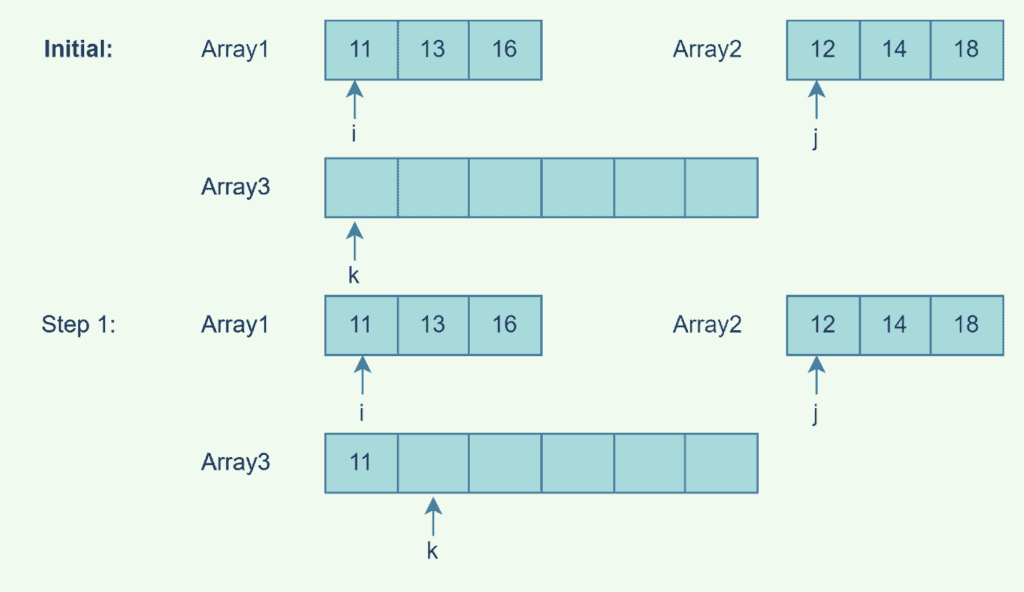We continue comparing and appending smaller items to the output array until the input arrays are empty: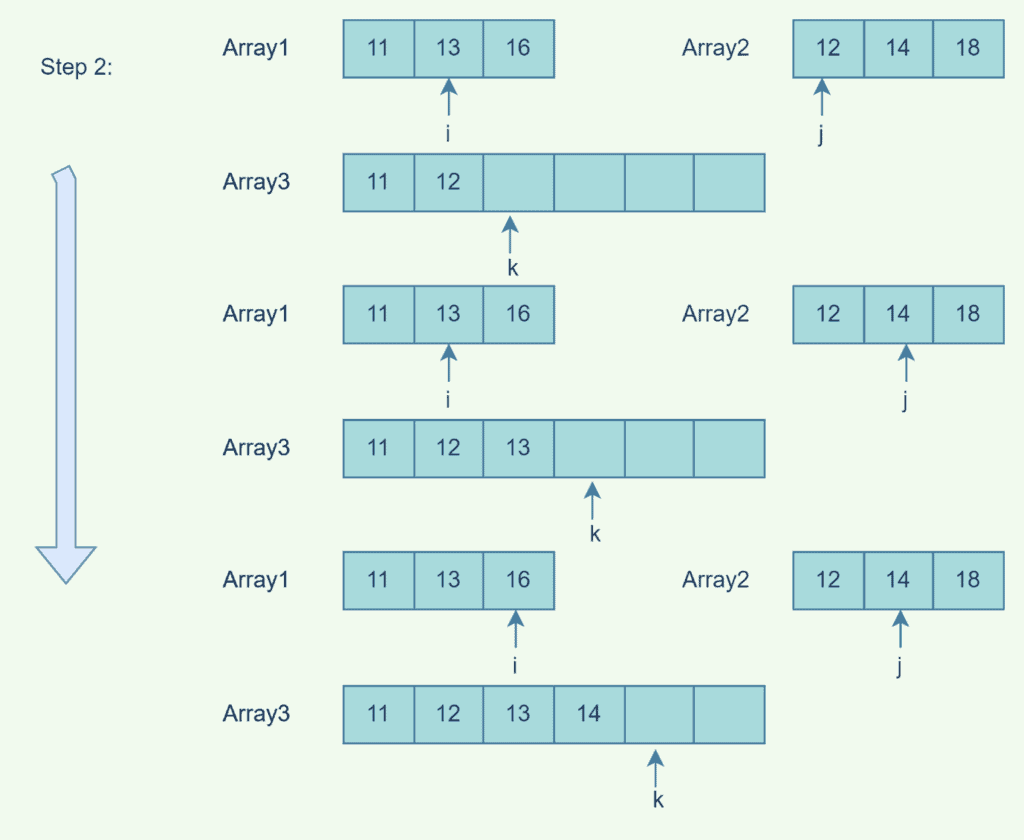At this point,is empty. We take the smaller item inand append it to the output since the other input array needs to be empty still. The process is repeated until: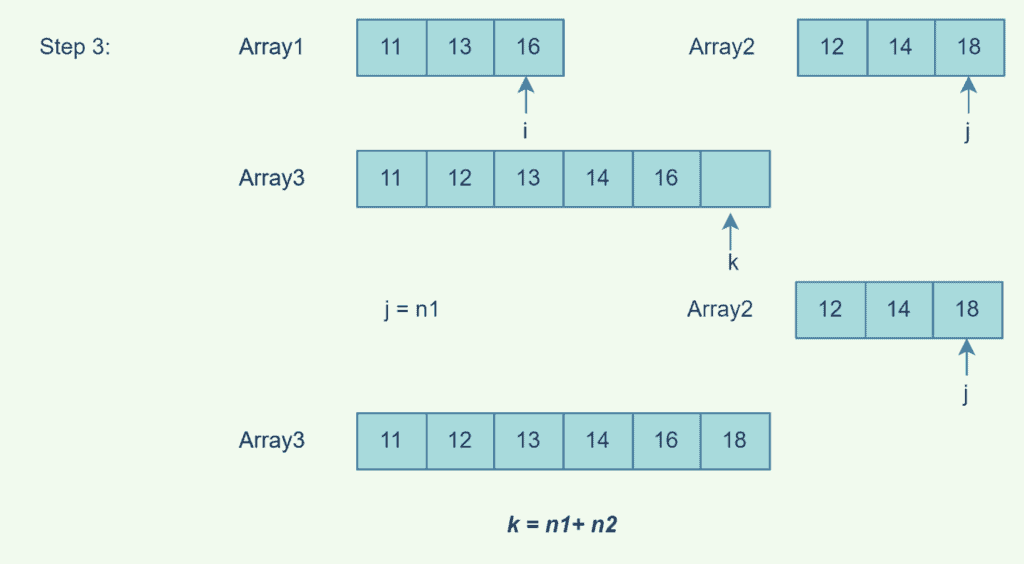### 2.2. End-to-End Merging

This type of merging simply merges one structure at the end of the other structure. Not to mention the structures that are merged can either be in sorted or unsorted order.

This technique simply appends one array to the end of another array, which can be either sorted or unsorted: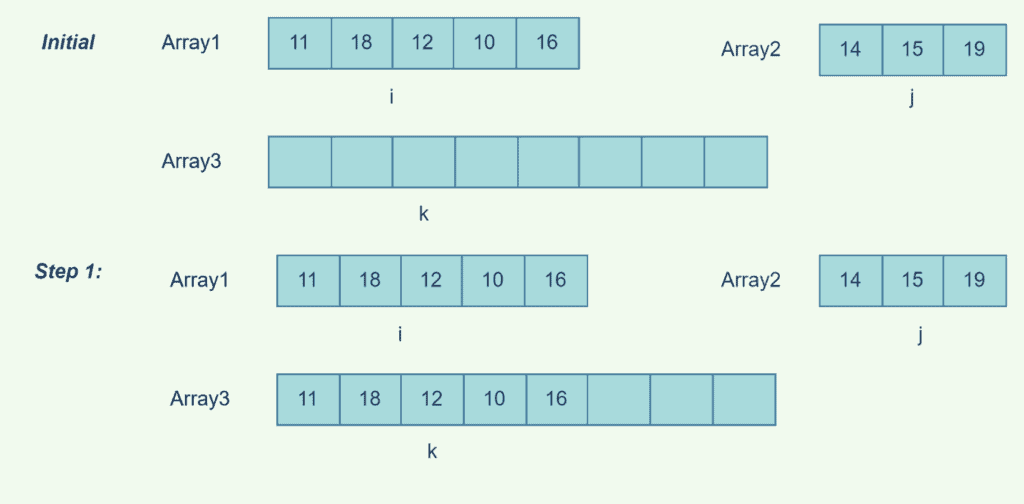In our case, we appendedtoand createdwith the same number of elements as the merged arrays: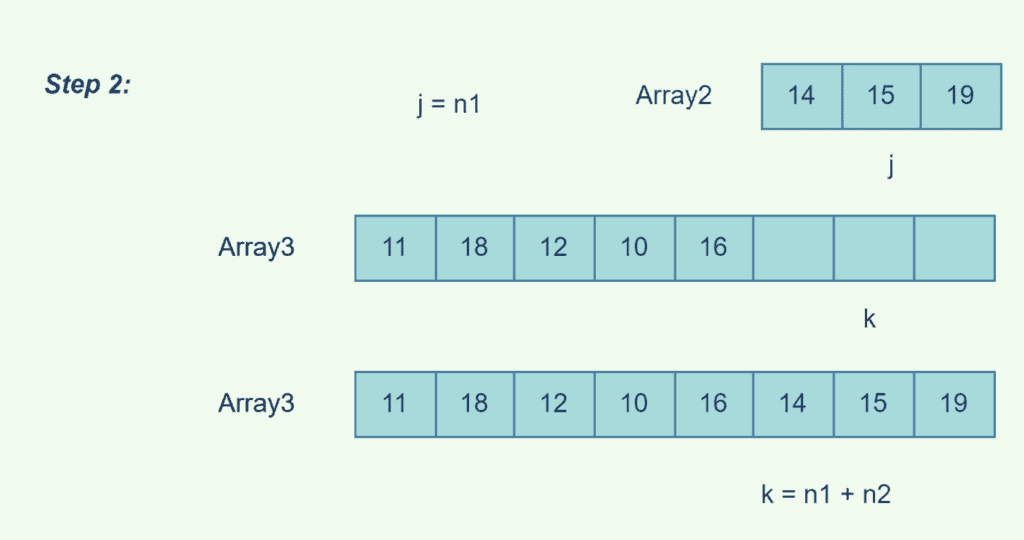## 3. Two-Way Merge

A two-way merging, also known as binary merging, is generally an algorithm that takes two sorted lists and merges them into one list in sorted order. It’s a widely used approach in merge sort that outputs the minimum item in each step given list in sorted order and builds a sorted list with all items in any of the input lists in proportion to the total of the lengths of the input lists.

If we are merging two arrays sizeandrespectively, then the merged array size will be the sum ofand. Furthermore, merging requires maximumcomparisons to get the merged array.

The detailed implementation of the two-way merge is shown in the diagrams below.

We compare the first items in two arrays in sorted order and then append the smaller element to the output array:After appending the smaller item to our output array, we continue comparing and appending them tountil the input arrays are empty:At this point,is empty. The other input array must still be empty, so we take the smaller item inand append it to. We repeat this process untilis empty.By comparing the first items in each input array and appending them to our output array, we obtained a merged sorted array with a size equal to the sum of the input array sizes.

Pseudocode for the two-way merging algorithm:

``

The 2-way merging algorithm works by comparing the first item of each list and moving the smallest one to the top of the output list.

In fact, this operation is repeated until one list is empty, at which point all entries of the second list are appended to the final output list.

The space complexity iswhen the complexity of time is.

## 4. K-Way Merge Algorithms-way merge algorithms, also known as multiway merges, are algorithms that take-sorted lists as input and produce one sorted list as an output with sizeequal to the sum of sizes of all input arrays.

The term “-way merge algorithms” refers to merging approaches that accept more than two sorted lists:

Pseudocode for the-way merging algorithm:

``

The detailed implementation of the– way merge is shown in the diagram below:

`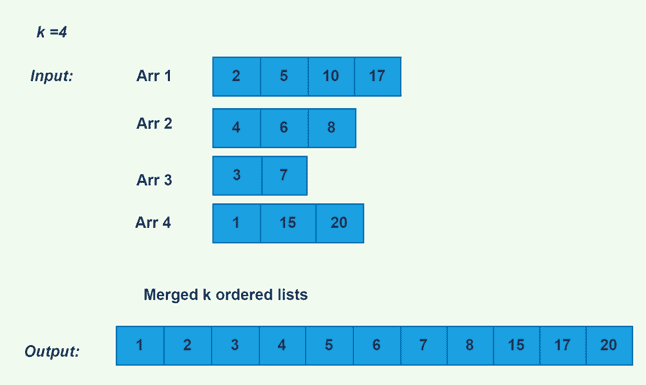`

We first create tournament trees by comparing the list’s indexitems and append the winner with the least value to the output list: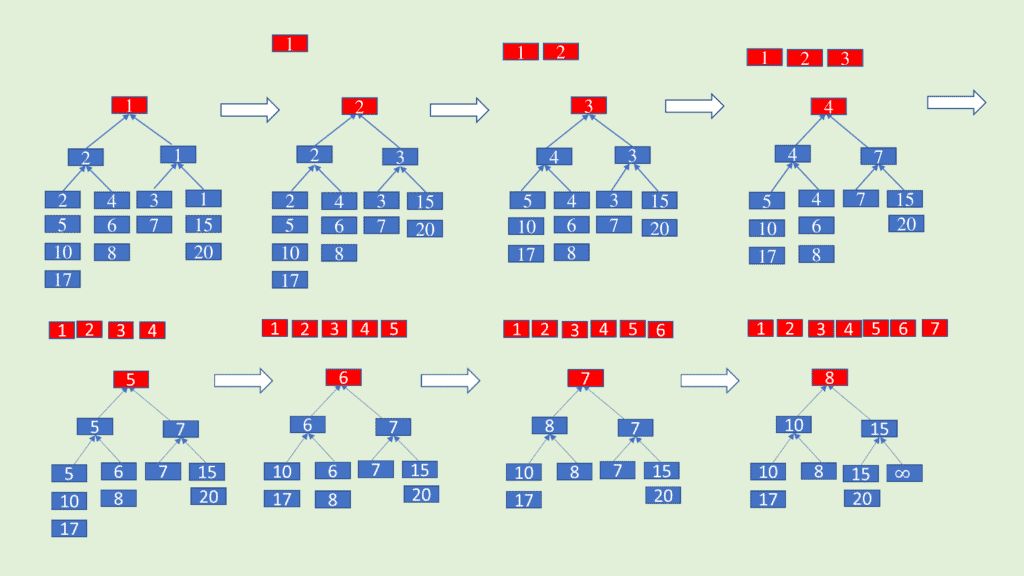We continue the process till the lists are empty: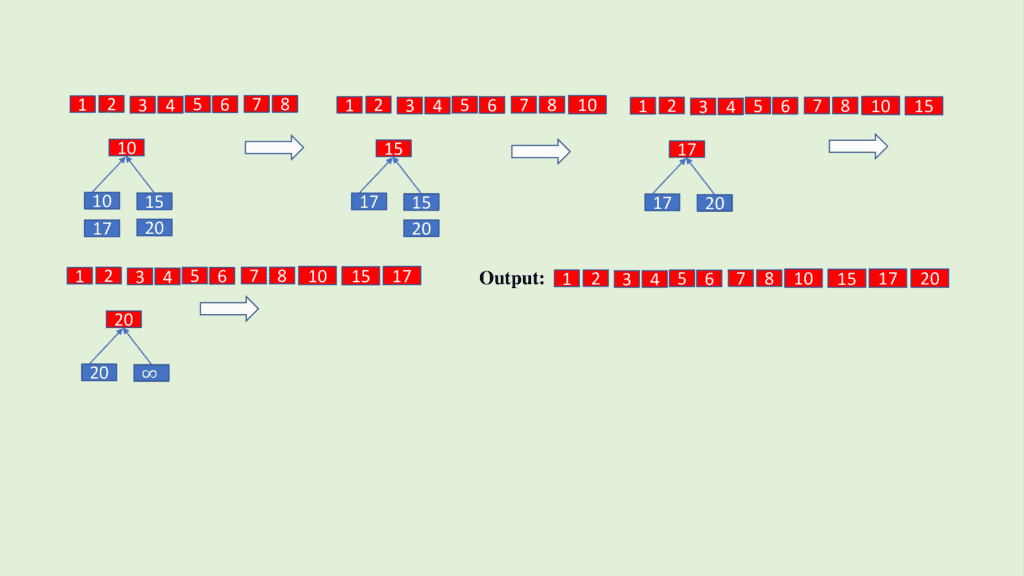In fact, the-way merging technique performs well if. Otherwise, determining the smallest value in each step would need a very large number of comparisons.

The space complexity is, while the time complexity is.

## 5. Conclusion

In this article, we defined the merging algorithms– Way Merge and Two Way Merge and discussed how they work. The complexity of merging algorithms has been explained in terms of both time and space. As a result, readers will have a better understanding of merging algorithms and their application areas.